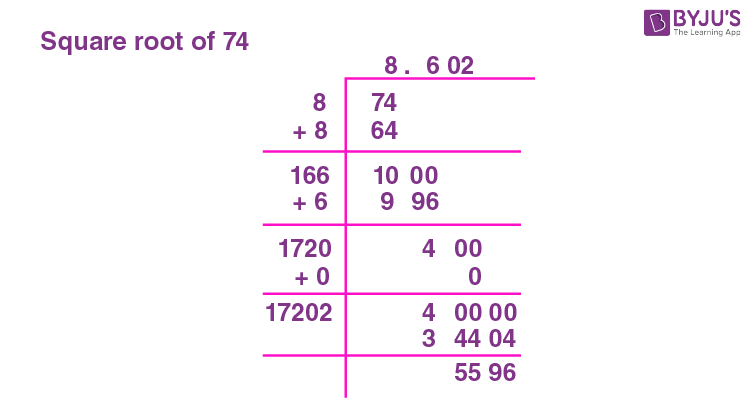# Square Root of 74

The square root of 74 is irrational. The approximate value of the square root of 74 is 8.6. When 8.6 is multiplied by 8.6, the product is 73.96, which when rounded off will be 74. Since 8.54 is multiplied by itself, 8.54 is the square root of 74. In general, when an integer “i” is multiplied with the same integer “i”, the resultant is called the perfect square and the integer “i” is its square root. i.e., If ± i × ± i = p, then i is the square root of p.

The given number 74 lies between 64 and 81, where 64 is obtained by the square of 8 ( ±8 × ±8 = 64) and 81 is obtained by multiplying 9 with 9. (±9 × ±9 = 81).

This means 74 is not a perfect square and does not have a perfect square root. Hence, the square root of 74,√74 is an irrational number. You can refer to Squares and Square roots for details.

Note the Following:

• The Square root of 74 = √74 = 2√74, where ‘√’ is radical, 74 is the radicand and 2 is the index
• Exponential Form of Square root of 74 = 741/2
• Solution for √74 ≈ 8.6
• The square root of 74 is Irrational = True

## What is the Square root of 74?

The square root of 74 is 8.6 as the square root of 74 is irrational.

 √74 =8.6

## How to Find the Square root of 74?

There are three methods to find the Square root of 74

• Prime Factorisation method
• Long Division method
• Repeated Subtraction method

### Square root of 74 by Prime Factorisation Method

In the prime factorization method, the following steps are followed.

1. Divide 74 with prime factors. Start with 2 as 74 is an even number.

The given number, 74 will be expressed as;

 2 74 37 37 × 1

To find the square root of 74 using the prime factorization method, grouping of the same factors is required. Since there are no common factors for grouping, the root of 74 cannot be determined using this method. Hence the square root of 74 is √74, which is irrational.

### Square root of 74 by Long Division Method

In the Long Division method, for 74, divide 74 by selecting a divisor such that d × d is less than or equal to 74. If d = 74, then d is the perfect square root of 74, it is irrational.

Choosing d from the list would be, the squares are:

8 × 8 = 64

9 × 9 = 81Hence 74 is not a perfect square. Therefore, 74 is an irrational number.

### Square root of 74 by Repeated Subtraction Method.

In the repeated Subtraction method, 74 is subtracted by odd numbers starting with 1, then 73 by 3, then 70 by 5 and so on. If these subtraction steps result in zero, then 74 will be a perfect square number, and the step at which zero is the remainder, its square root.

The table below shows repeated subtraction for the given number 74.

 Step 1 74 – 1 = 73 Step 2 73 – 3 = 70 Step 3 70 – 5 = 65 Step 4 65 – 7 = 58 Step 5 58 – 9 = 49 Step 6 49 – 11 = 38 Step 7 38 – 13 = 25 Step 8 25 – 15 = 10 Step 9 10 – 17 = -7

Since the difference becomes negative at step 9, from the positive number at step 8, root of 74 lies between 8 and 9. Therefore, 74 is not a perfect square number and does not have a perfect square root.

## Video Lessons

### Visualising square roots### Finding Square roots## Solved Examples

1. Which number greater than 74 is a perfect square root?

Solution: 81, which is greater than 74, is a perfect square number.

2. What is the square root of 64?

Solution: 8 is the square root of 64.

## Frequently Asked Questions on Square root of 74

### What is the Square root of 74?

The Square root of 74 is 8.6

### 74 is a perfect square number. True or False?

False. 74 is not a perfect square number.

### What are the factors of 74?

The factors of 74 are 1, 2, 37 and 74.

### Simplify √74?

√74 = √(2 × 37). No further simplification is possible.

### The square root of 74 is a rational number. True or False?

False. The square root of 74 is not a rational number.